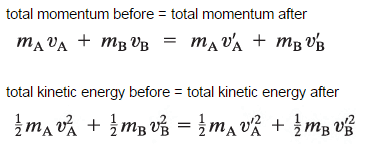# Isolating Variable in Equation for conservation of momentum

• jxj
No, you want a single ##m_\mathrm{A}##. You need to do the inverse of distribution:$$a (b+c) = ab +...+ ac #### jxj ## Homework Statement So the problem is trying to isolate mAin the equation for momentum (only focusing on top formula, not bottom hehe) basically by solving the equation I assume. My teacher said because the vA and vB on the right were prime they could not be combined so I'm having trouble computing it. Please help! ## Homework Equations## The Attempt at a Solution am pretty lost. I believe you have to divide the primes? I am unsure #### Attachments Start by putting all terms containing ##m_\mathrm{A}## on the left-hand side, and all other terms on the right-hand side. •jxj jxj said: My teacher said because the vA and vB on the right were prime they could not be combined You may be misinterpreting that. Ignore it and see where you get to. •jxj DrClaude said: Start by putting all terms containing ##m_\mathrm{A}## on the left-hand side, and all other terms on the right-hand side. when doing this, don't you divide the ##m_\mathrm{A}## plus ##v_\mathrm{A}## prime on the right by its counterpart on the left first? even though it contains a prime? haruspex said: You may be misinterpreting that. Ignore it and see where you get to. ok thanks jxj said: when doing this, don't you divide the ##m_\mathrm{A}## plus ##v_\mathrm{A}## prime on the right by its counterpart on the left first? No, I wouldn't divide yet, I would simply start by sorting the terms. When you have an equation like$$
5x -3 = 2x + 1
$$the first step is to try and gather all terms in ##x## together, and leave the rest on the other side,$$
\begin{align*}
5x - 3 -2x +3 &= 2x + 1 - 2x + 3 \\
3x &= 4
\end{align*}
$$DrClaude said: No, I wouldn't divide yet, I would simply start by sorting the terms. When you have an equation like$$
5x -3 = 2x + 1
$$the first step is to try and gather all terms in ##x## together, and leave the rest on the other side,$$
\begin{align*}
5x - 3 -2x +3 &= 2x + 1 - 2x + 3 \\
3x &= 4
\end{align*}
$$ok so right now I am a little confused because unlike the example you gave with 5x, the formula is essentially equal on both sides minus the primes. in my mind I would try to cross out the mAvA on the right, is that right? DrClaude said: No, I wouldn't divide yet, I would simply start by sorting the terms. When you have an equation like$$
5x -3 = 2x + 1
$$the first step is to try and gather all terms in ##x## together, and leave the rest on the other side,$$
\begin{align*}
5x - 3 -2x +3 &= 2x + 1 - 2x + 3 \\
3x &= 4
\end{align*}
$$I tried putting all of mA on the left and I got to mAvA - mAvA (prime) = mBvB (prime) - mBvB did I mess up? jxj said: I tried putting all of mA on the left and I got to mAvA - mAvA (prime) = mBvB (prime) - mBvB did I mess up? No, that's correct. How you want to think of it is doing the same thing to both sides. So to move "m_a v'_a" to the left, subtract it from both sides. Or if it was multiplied by that, you would divide it on both sides. •jxj jxj said: I tried putting all of mA on the left and I got to mAvA - mAvA (prime) = mBvB (prime) - mBvB did I mess up? Factorise each side. •jxj haruspex said: Factorise each side. when you say factorise could you explain a little more please jxj said: when you say factorise could you explain a little more please You want to go from ##m_\mathrm{A} v_\mathrm{A} - m_\mathrm{A} v_\mathrm{A}'## to an expression where ##m_\mathrm{A}## appears only once, using the distributive property of multiplication. DrClaude said: You want to go from ##m_\mathrm{A} v_\mathrm{A} - m_\mathrm{A} v_\mathrm{A}'## to an expression where ##m_\mathrm{A}## appears only once, using the distributive property of multiplication. thanks! DrClaude said: You want to go from ##m_\mathrm{A} v_\mathrm{A} - m_\mathrm{A} v_\mathrm{A}'## to an expression where ##m_\mathrm{A}## appears only once, using the distributive property of multiplication. so right now I am at distributing mA by itself into (vA - mAv’A) on the left of the equal sign and vice versa for mB on the right of the equal sign. am I on the right track? jxj said: so right now I am at distributing mA by itself into (vA - mAv’A) on the left of the equal sign and vice versa for mB on the right of the equal sign. Check that part I highlighted in red. •jxj DrClaude said: Check that part I highlighted in red. for that part, was I supposed to separate them so I would have mA(vA) -(mA)(v’A) ? jxj said: for that part, was I supposed to separate them so I would have mA(vA) -(mA)(v’A) ? No, you want a single ##m_\mathrm{A}##. You need to do the inverse of distribution:$$
a (b+c) = ab + ac


•jxj